# The Number of Clusters

There are no completely satisfactory methods that can be used for determining the number of population clusters for any type of cluster analysis (Everitt; 1979; Hartigan; 1985a; Bock; 1985).

If your purpose in clustering is dissection—that is, to summarize the data without trying to uncover real clusters—it might suffice to look at R square for each variable and pooled over all variables. Plots of R square against the number of clusters are useful.

It is always a good idea to look at your data graphically. If you have only two or three variables, use PROC SGPLOT to make scatter plots identifying the clusters. With more variables, use PROC CANDISC to compute canonical variables for plotting.

Ordinary significance tests, such as analysis of variance F tests, are not valid for testing differences between clusters. Since clustering methods attempt to maximize the separation between clusters, the assumptions of the usual significance tests, parametric or nonparametric, are drastically violated. For example, if you take a sample of 100 observations from a single univariate normal distribution, have PROC FASTCLUS divide it into two clusters, and run a t test between the clusters, you usually obtain a p-value of less than 0.0001. For the same reason, methods that purport to test for clusters against the null hypothesis that objects are assigned randomly to clusters (such as McClain and Rao 1975 and Klastorin 1983) are useless.

Most valid tests for clusters either have intractable sampling distributions or involve null hypotheses for which rejection is uninformative. For clustering methods based on distance matrices, a popular null hypothesis is that all permutations of the values in the distance matrix are equally likely (Ling; 1973; Hubert; 1974). Using this null hypothesis, you can do a permutation test or a rank test. The trouble with the permutation hypothesis is that, with any real data, the null hypothesis is implausible even if the data do not contain clusters. Rejecting the null hypothesis does not provide any useful information (Hubert and Baker; 1977).

Another common null hypothesis is that the data are a random sample from a multivariate normal distribution (Wolfe; 1970, 1978; Duda and Hart; 1973; Lee; 1979). The multivariate normal null hypothesis arises naturally in normal mixture models (Titterington, Smith, and Makov; 1985; McLachlan and Basford; 1988). Unfortunately, the likelihood ratio test statistic does not have the usual asymptotic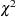distribution because the regularity conditions do not hold. Approximations to the asymptotic distribution of the likelihood ratio have been suggested Wolfe (1978), but the adequacy of these approximations is debatable (Everitt; 1981; Thode, Mendell, and Finch; 1988). For small samples, bootstrapping seems preferable (McLachlan and Basford; 1988). Bayesian inference provides a promising alternative to likelihood ratio tests for the number of mixture components for both normal mixtures and other types of distributions (Binder; 1978, 1981; Banfield and Raftery; 1993; Bensmail et al.; 1997).

The multivariate normal null hypothesis is better than the permutation null hypothesis, but it is not satisfactory because there is typically a high probability of rejection if the data are sampled from a distribution with lower kurtosis than a normal distribution, such as a uniform distribution. The tables in Englemann and Hartigan (1969), for example, generally lead to rejection of the null hypothesis when the data are sampled from a uniform distribution. Hawkins, Muller, and ten Krooden (1982, pp. 337–340) discuss a highly conservative Bonferroni method for the use of hypothesis testing. The conservativeness of this approach might compensate to some extent for the liberalness exhibited by tests based on normal distributions when the population is uniform.

Perhaps a better null hypothesis is that the data are sampled from a uniform distribution (Hartigan; 1978; Arnold; 1979; Sarle; 1983) The uniform null hypothesis leads to conservative error rates when the data are sampled from a strongly unimodal distribution such as the normal. However, in two or more dimensions and depending on the test statistic, the results can be very sensitive to the shape of the region of support of the uniform distribution. Sarle (1983) suggests using a hyperbox with sides proportional in length to the singular values of the centered coordinate matrix.

Given that the uniform distribution provides an appropriate null hypothesis, there are still serious difficulties in obtaining sampling distributions. Some asymptotic results are available (Hartigan; 1978, 1985a; Pollard; 1981; Bock; 1985) for the within-cluster sum of squares, the criterion that PROC FASTCLUS and Ward’s minimum variance method attempt to optimize. No distributional theory for finite sample sizes has yet appeared. Currently, the only practical way to obtain sampling distributions for realistic sample sizes is by computer simulation.

Arnold (1979) used simulation to derive tables of the distribution of a criterion based on the determinant of the within-cluster sum of squares matrix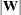. Both normal and uniform null distributions were used. Having obtained clusters with either PROC FASTCLUS or PROC CLUSTER, you can compute Arnold’s criterion with the ANOVA or CANDISC procedure. Arnold’s tables provide a conservative test because PROC FASTCLUS and PROC CLUSTER attempt to minimize the trace of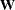rather than the determinant. Marriott (1971, 1975) also provides useful information aboutas a criterion for the number of clusters.

Sarle (1983) used extensive simulations to develop the cubic clustering criterion (CCC), which can be used for crude hypothesis testing and estimating the number of population clusters. The CCC is based on the assumption that a uniform distribution on a hyperrectangle will be divided into clusters shaped roughly like hypercubes. In large samples that can be divided into the appropriate number of hypercubes, this assumption gives very accurate results. In other cases the approximation is generally conservative. For details about the interpretation of the CCC, consult Sarle (1983).

Milligan and Cooper (1985) and Cooper and Milligan (1988) compared 30 methods of estimating the number of population clusters by using four hierarchical clustering methods. The three criteria that performed best in these simulation studies with a high degree of error in the data were a pseudo F statistic developed by Calinski and Harabasz (1974), a statistic referred to as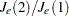by Duda and Hart (1973) that can be transformed into a pseudo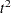statistic, and the cubic clustering criterion. The pseudo F statistic and the CCC are displayed by PROC FASTCLUS; these two statistics and the pseudostatistic, which can be applied only to hierarchical methods, are displayed by PROC CLUSTER. It might be advisable to look for consensus among the three statistics—that is, local peaks of the CCC and pseudo F statistic combined with a small value of the pseudostatistic and a larger pseudofor the next cluster fusion. It must be emphasized that these criteria are appropriate only for compact or slightly elongated clusters, preferably clusters that are roughly multivariate normal.

Recent research has tended to deemphasize mixture models in favor of nonparametric models in which clusters correspond to modes in the probability density function. Hartigan and Hartigan (1985) and Hartigan (1985b) developed a test of unimodality versus bimodality in the univariate case.

Nonparametric tests for the number of clusters can also be based on nonparametric density estimates. This approach requires much weaker assumptions than mixture models, namely, that the observations are sampled independently and that the distribution can be estimated nonparametrically. Silverman (1986) describes a bootstrap test for the number of modes using a Gaussian kernel density estimate, but problems have been reported with this method under the uniform null distribution. Further developments in nonparametric methods are given by Müller and Sawitzki (1991), Minnotte (1992), and Polonik (1993). All of these methods suffer from heavy computational requirements.

One useful descriptive approach to the number-of-clusters problem is provided by Wong and Schaack (1982) based on a kth-nearest-neighbor density estimate. The kth-nearest-neighbor clustering method developed by Wong and Lane (1983) is applied with varying values of k. Each value of k yields an estimate of the number of modal clusters. If the estimated number of modal clusters is constant for a wide range of k values, there is strong evidence of at least that many modes in the population. A plot of the estimated number of modes against k can be highly informative. Attempts to derive a formal hypothesis test from this diagnostic plot have met with difficulties, but a simulation approach similar to Silverman (1986) does seem to work Girman (1994). The simulation, of course, requires considerable computer time.

PROC MODECLUS uses a less expensive approximate nonparametric test for the number of clusters. This test sacrifices statistical efficiency for computational efficiency. The method for conducting significance tests is described in the chapter on the MODECLUS procedure. This method has the following useful features:

• No distributional assumptions are required.

• The choice of smoothing parameter is not critical since you can try any number of different values.

• The data can be coordinates or distances.

• Time and space requirements for the significance tests are no worse than those for obtaining the clusters.

• The power is high enough to be useful for practical purposes.

The method for computing the p-values is based on a series of plausible approximations. There are as yet no rigorous proofs that the method is infallible. Neither are there any asymptotic results. However, simulations for sample sizes ranging from 20 to 2000 indicate that the p-values are almost always conservative. The only case discovered so far in which the p-values are liberal is a uniform distribution in one dimension for which the simulated error rates exceed the nominal significance level only slightly for a limited range of sample sizes.